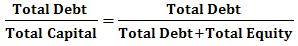# Debt to Capital Ratio

Debt to Capital Ratio is a type of Solvency Ratio that determines the contribution of Debt in a company’s Total Capital. It is a measure of the total debt of a company relative to its total capital. Creditor's contribution is termed as Debt and Debt along with total Equity is termed as Total Capital.
$$Debt\quad to\quad Capital\quad Ratio=\frac { Total\quad Debt }{ Total\quad Capital }$$Total Debt = Long Term Debt + Current or Short-Term Debt; hence, total debt includes all debts/liability payable within a year and payable in the long-term.
Where,
• And Total Capital = Total Equity + Total Debt; total capital implies shareholders as well as creditors contribution.

## Significance and Interpretation

• Debt to Capital Ratio =• For, Debt to Capital Ratio = 1, Total Equity must be Zero, which is not possible.
• Hence, the Maximum Value of Debt Capital Ratio = 1
• Debt Capital Ratio = 0.5: This implies that the Debt Contribute to 50% of the Total Capital or Total Debt is equal to Total Equity i.e. there is just enough Equity to cover all Debt.
• Debt Capital Ratio <0.5: This implies that Debt contributes to less than 50% of the total capital and there is enough equity to cover all debts.
• Debt Capital Ratio > 0.5: This implies that Debt contributes to more than 50% of the total capital, the company faces lots of issues in times when the interest rates rise.
• The ideal Debt Capital Ratio < 0.5, which indicates that the company has less than half of the capital as debt (both current as well as non-current). However, it must be noted that this limit may shift depending upon the regulatory reforms and/or type of business.
• A low Debt to Capital Ratio is beneficial for lenders to the company, wherein a high Debt to Capital Ratio is beneficial to the company for trading in Equities.

### Examples

#### Example 1:

M/S ABC Ltd. reported short term debts worth ₹80 Crores, long term debts worth ₹ 220 Crores and total equity as ₹450 Crores, find the debt capital ratio of M/S ABC Ltd

#### Solution:

Total Debt = Short Term Debt + Long Term Debt
(80+220)
₹300 Crore
Total Capital = Total Debt + Total Equity
(300 + 450)
₹750 Crore
Hence, Debt Capital Ratio = Total Debt / Total Capital
300/750
2/5 or 0.4

#### Example 2:

The following information is available about M/S XYZ Ltd, find debt capital ratio of the firm.
Sr. No Particulars Amount (in ₹ Cr)
1 Current Liability 400.00
2 Non-Current liability 120.00
3 Share Capital 180.00
4 Money Reserved Against Share Warrants 800.00
5 Reserves and Surplus 50.00

#### Solution:

Total Debt = Current Liability + Non- Current Liability
₹520Crore
Total Capital = Total Debt + Share Capital + Money Reserved Against Share Warrants + Reserves and Surplus
₹1030 Crore
Debt Capital Ratio = Total Debt / Total Capital
520/1030
52/103
Hence, Debt Capital Ratio = 52/103 or 0.5